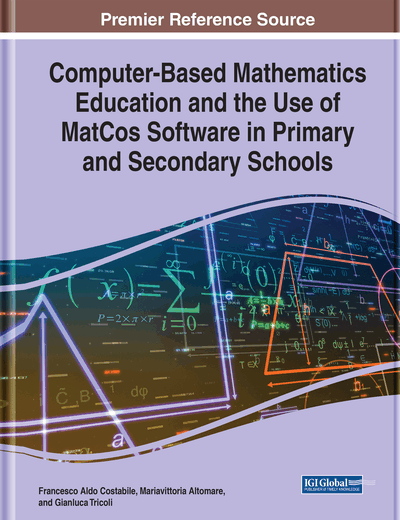# Activities in the Secondary School: A Geometric Path

DOI: 10.4018/978-1-7998-5718-1.ch007

## Abstract

With the same methodology of the previous chapter, in this chapter there is an outline of vertical path about geometric topics typical of the secondary school. Of course, the algorithm and computational aspect in the MatCos 3.X environment are more developed with respect to classical arguments, surely of interest. In particular, the presentation of conics in both the Euclidean and Cartesian plan is emphasized, based on construction algorithms by points, which can be easily implemented in the MatCos 3.X programming environment. Even solid geometry, or in three dimensions, will be characterized by effective construction algorithms of the solid figures presented. Some of these algorithms are general in nature.
Chapter Preview

“…the original works of the forerunners of Euclide, Archimede and Apollonius are lost, having probably been discarded and forgotten almost immediately after the appearance of the masterpieces of that great trio.” ~T. L. Heath,1926, vol I,p.29

Like the geometer, who gives himself wholly to measuring the circle, nor, by thinking, finds the principle he needs; ev’n such was I at that new sight. ~Dante Alighieri, Divina Commedia, Translate: Courtney Langdon

Top

## 2. Point, Line, Segment, Straight-Line, Half-Line: A Reminder With Some Insights.

In the primary school we have already met the fundamental geometric entities of plane geometry, in this brief reference we mean to emphasize the abstract nature of the geometric entities, differentiating them from their visual or interpretative representation in the reality that surrounds us. For example, the following MatCos 3.X commands represent points that would appear to be different in size and shape, but as geometric entities they represent only the geometric object Point. The following genetic and axiomatic commands draw points of different sizes and colours:

• PenColor(0,0,255);

• PenWidth(1);

• Point;

• PenWidth(6);

• Point;

So, the Point is an abstract geometric entity, whose image in the real-world can be the sign left by a pencil on a sheet of paper, as well as the mark left by the mouse on the screen, regardless of its shape (circular, square, etc.) and size. In fact, in a possible mathematical modelling a point can represent a cell in a culture of bacteria, but a point can also represent a large ship on the ocean.

## Key Terms in this Chapter

Mathematic Modelling: Is the art of translating problems from an application area into tractable mathematical formulations whose theoretical and numerical analysis provides insight, answers, and guidance useful for the originating application.

Plane Geometry: Geometry on two dimensions.

Platonic Solid: Is a regular, convex polyhedron.

Rotation Solid: A solid figure obtained by rotating a plane curve around some straight line (the axis of revolution) that lies on the same plane.

MatCos 3.X: Programming environment oriented towards mathematics, aimed at high school.

TLS: Acronym of “teaching learning sequence.”

Solid Geometry: Geometry on three dimensions.

## Complete Chapter List

Search this Book:
Reset